# A Certain Uncertainty: The Heisenberg Uncertainty Principle Explained

by Robert Lea 2 years ago in science

The Heisenberg uncertainty principle doesn’t just underpin the foundations of quantum mechanics — it also explains phenomena across science such as the stability of stars, and the phenomenon of electric currents.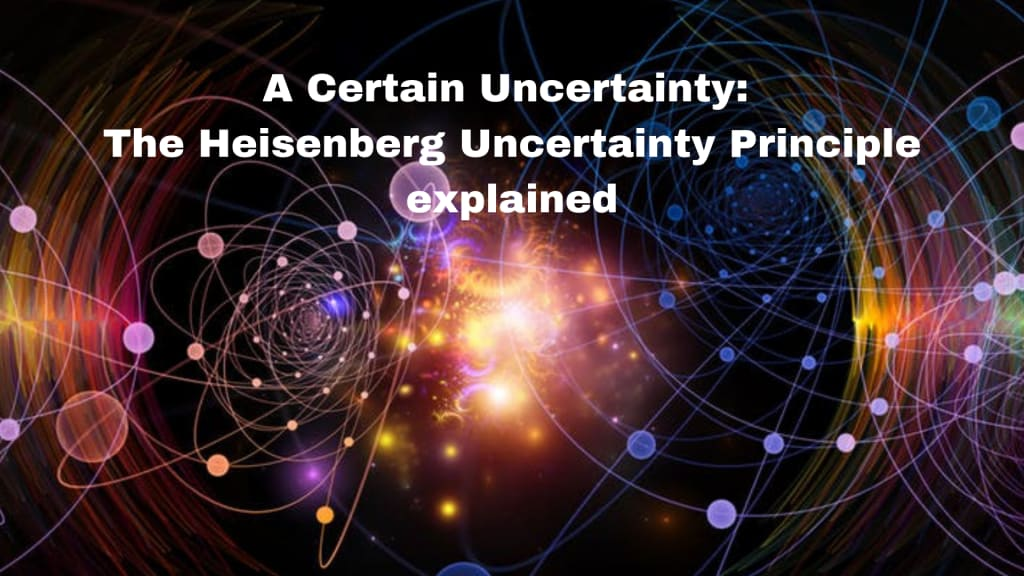'Uncertainty'—it's not a word that we generally associate with science. Science conjures images of certainty, solid results, and constant improvement by increment. There's a certain irony then, that when we strip nature down to its fundamental limits, there remains an implicit, irremovable, intrinsic uncertainty. No matter how precise our measuring devices and methods become, no matter how clever our scientists, nature has imposed a limit on what we can know. And we are certain of that. That's what the Heisenberg Uncertainty Principle describes. And it isn't just a factor in quantum physics. It infiltrates our lives in a number of surprising ways.

## How the Uncertainty Principle Became a Pop-Culture Sensation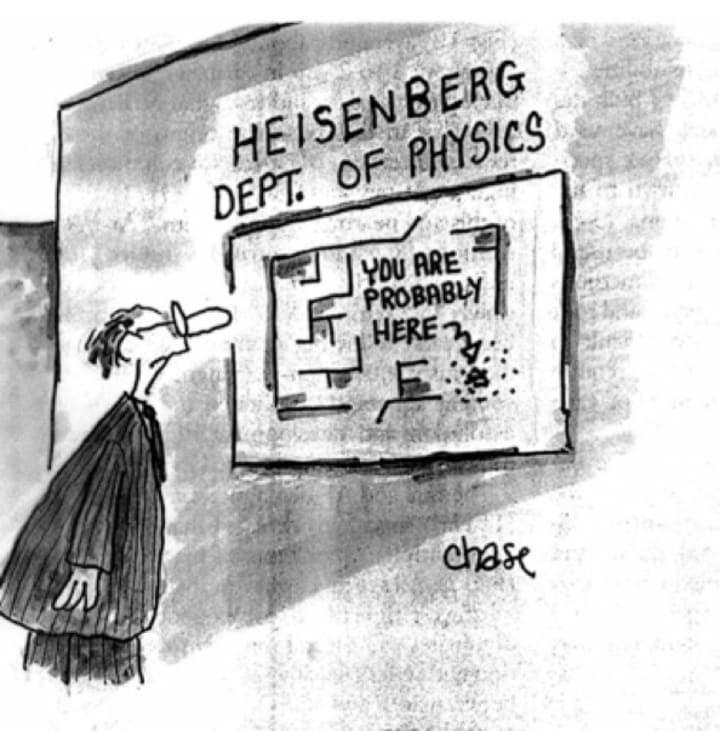There's no doubt, quantum physics has captured the imagination of the public since its inception at the turn of the 19th century—unsurprising given how counter-intuitive it can be. This adds a sense of mystery to the discipline. An element missing in other scientific pursuits.

Because of this interest, certain elements from quantum physics have escaped dry physics textbooks and lectures, and passed into the public domain. Concepts like Schrodinger’s cat, particle-wave duality, and the many worlds interpretation of quantum mechanics appear in newspaper cartoons, on mugs and witty tee-shirts. These concepts also appear frequently in news stories, magazine articles, and television shows. Unfortunately, when these concepts do crop up in the media they are frequently poorly explained to the reader, or are misunderstood by the author.

The Heisenberg Uncertainty Principle is a prime example of this. First introduced in 1927 by Werner Heisenberg towards the end of a flurry of discoveries that opened the microscopic world up to physicists, the uncertainty principle is without a doubt, one of the most celebrated results in all of physics, and thus as misunderstood as any other facet of quantum physics.

## What Is the Heisenberg Uncertainty Principle?

The uncertainty principle states that there is an intrinsic limit to the precision with which we can simultaneously know two complementary variables of a quantum system. For example, the concepts of exact position, and exact velocity together have no meaning in nature. The more precisely that you know the position of a particle, the less precisely you can know its momentum and vice versa.

The idea of this may sound somewhat mundane, but consider that it forces physicists to abandon the very concept of the trajectory of a particle on a quantum scale. It also underpins and explains elements of physics that most of us find completely mind-boggling, such as the idea of particles tunneling through solid barriers without the necessary kinetic energy, and the idea that a pair of virtual particles can appear from the vacuum of space, but only for a limited time.

Consider this analogy between classical physics and quantum physics: Imagine tossing a “classical” ball into the air, we know its mass and its velocity, so we can map its precise trajectory, determine where it will land, and crucially, the point where its kinetic energy will have transformed fully into gravitational potential energy, and its ascent will stop. For a split second, it halts in the air. Then it falls, gradually exchanging its potential energy for kinetic energy.

Now let’s throw our quantum mechanical ball into the air. Again, we know precisely its mass and the velocity at which it travels. As a result of this knowledge, the Heisenberg uncertainty principle tells us that we must consider there to be a finite possibility that the quantum ball will continue to travel after its peak. This is because we are forbidden to know precisely where the ball will stop for that split second when its kinetic energy is exhausted. Thus, there’s a probability of finding our quantum ball in a region that is classically forbidden.

Not so mundane anymore.

## Not just the observer effect...

Commonly, the Heisenberg uncertainty principle is confused with the observer or experimenter effect. This states that the act of attempting to measure the observables (such as momentum, position, and energy) of a system fundamentally changes the state of this system. A straightforward way to think of this at a macroscopic scale is by considering that electrons change energy levels by emitting and observing photons. The only way to “observe” an electron is to bombard it with photons, thus any attempt to measure electrons fundamentally changes the state of those electrons.

The uncertainty principle is also often conflated with the idea that all scientific experiments have an experimental uncertainty, which depends on the sensitivity of the measuring equipment used. But, unlike the experimenter effect, the Heisenberg uncertainty principle does not tell us that a measurement of position affects momentum or vice versa. The Heisenberg uncertainty principle is much deeper than this; no matter how accurate our measuring equipment becomes, no matter how careful and diligent our researchers, how well composed our experiments, the Heisenberg uncertainty principle remains as a fundamental limit to our knowledge. Almost as if nature is telling us that we can’t have our cake and eat it too.

## Where does the Heisenberg Uncertainty Principle come from?

The relationship between the momentum and position of a particle is the form of the Heisenberg uncertainty principle, and possibly the easiest to explain. Now is an ideal time to introduce and explain the inequality that underpins the Heisenberg uncertainty principle.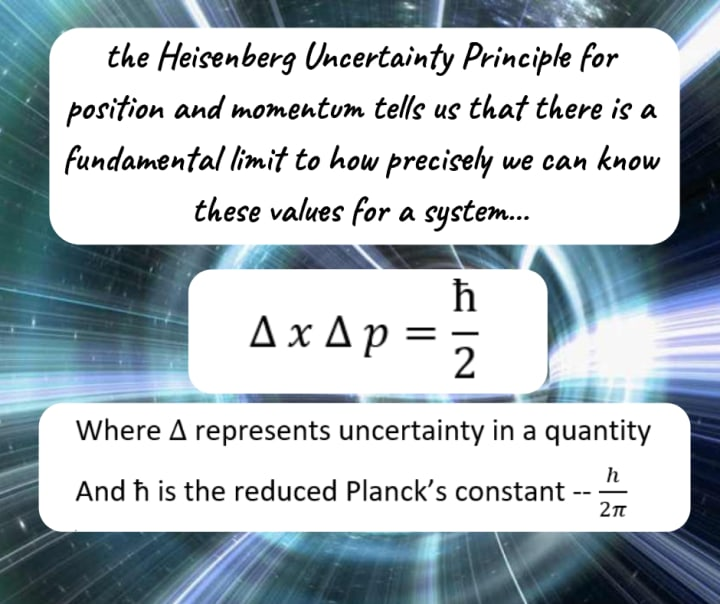The first thing you’ll notice is this isn’t an equation, it lacks an equals sign. The left-hand side is linked to the right-hand side by a symbol that tells us the left-hand side of the inequality is free to take any value as long as it is greater than or equal to the right-hand side.

The deltas—represented by triangles—in the above inequality represent uncertainty, so delta x is our uncertainty in position. Delta p is our uncertainty in momentum.

Putting this together tells us the product of our uncertainty in position, multiplied by our uncertainty in momentum must be greater than Planck’s constant (h-bar) over 2 — a very small number indeed as Planck’s constant itself is so small. In fact, it’s the tiny magnitude of Planck’s constant that explains why quantum effects aren’t seen in our everyday world. The scale on which we measure macroscopic objects is so large in comparison to Planck’s constant, that the left-hand side of our inequality would always dwarf the left-hand side, which is of course perfectly allowed by the uncertainty principle.

So, next time you forget where you parked, you cannot claim that your lack of knowledge of the car’s position is due to the fact you are reasonably sure of its momentum.

Fundamentally, the Heisenberg uncertainty principle arises from the fact that we can define matter as exhibiting both wave-like and particle-like behaviour. Momentum is given by the equation below, where the denominator on the right-hand side is the wavelength of the wave in question.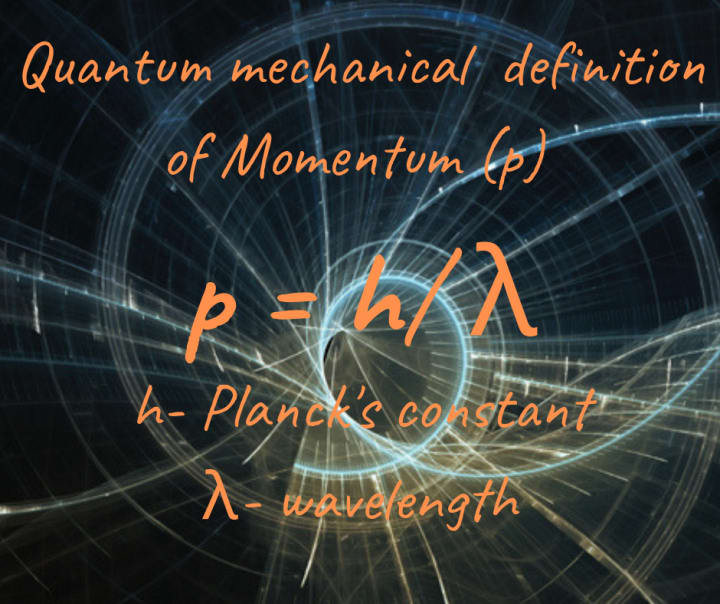## Thus, momentum is intrinsically tied to wavelength, usually defined as the peak of one crest to another.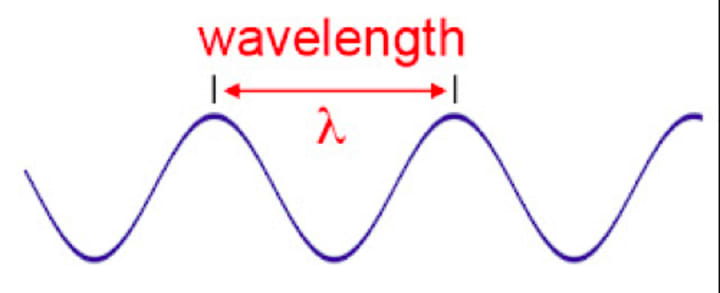A perfect wave description of momentum would look something like the image below where the x-axis is the location and the y-axis is the probability of finding the particle at this location, the graph forms an infinitely long sine wave.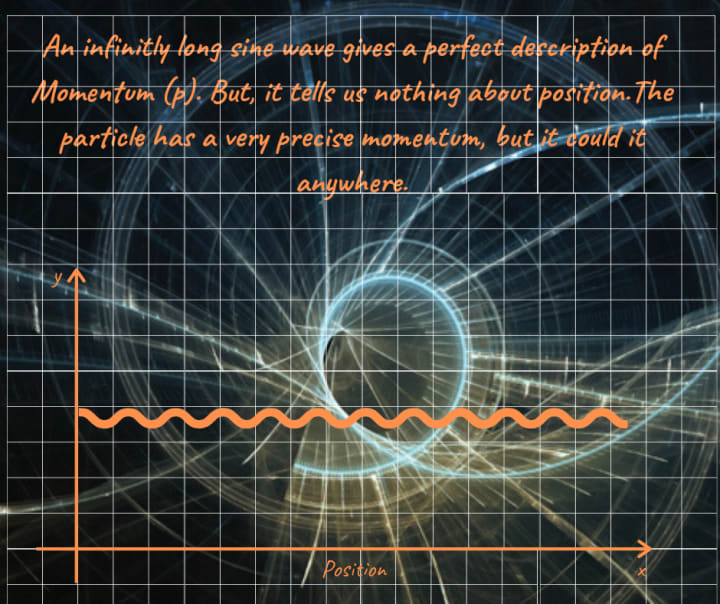So let's see what a graphical representation of a precisely located particle looks like.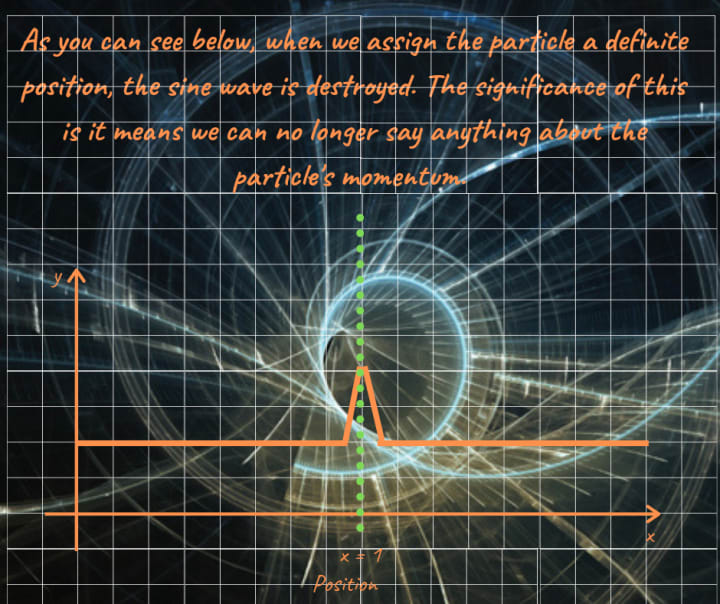The probability of finding a particle anywhere but at x=1 is zero. But the wavelength is gone, and thus the momentum is no longer ascertainable. This is apt physical proof of this form of the Heisenberg uncertainty principle, but what are the actual effects of the Heisenberg uncertainty principle in our everyday world?

In short, our very existence is a result of a phenomenon built on this aspect of nature.

## The Heisenberg Uncertainty Principle and Quantum Tunnelling

A startling display of the non-intuitive nature of quantum physics is the concept of barrier penetration. Imagine a particle of certain energy <E> approaching a barrier, which can be defined in terms of potential energy. If the particle’s kinetic energy is greater than this potential energy we should expect the particle to safely clear the barrier, but what if the particle has insufficient energy?

Classical physics would tell us that the area beyond the barrier is a “forbidden zone” in which we will never find the particle. The predictions of quantum mechanics are quite different however, and have been confirmed experimentally many times. The images taken from a simulation program below show this in effect. In the simulation, we set the barrier’s potential energy at 150eV, and the particle’s kinetic energy at 104eV.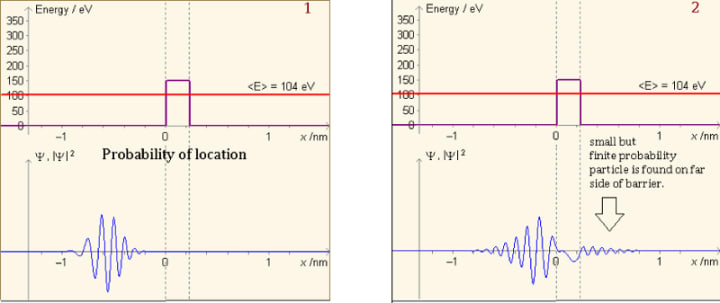As you can see there is a small but finite probability of finding the particle on the far side of the barrier.

This is similar to the “quantum ball” example set at the top of the article, the uncertainty principle doesn’t allow sharp turning points. There’s a “fuzziness” to outcomes at a quantum level. The amazing aspect of this is that when this experiment is carried out in the lab, particles found in the forbidden region have the same kinetic energy that they had on their approach. This tells us that the particles aren’t “punching through” the barrier, as this would cause them to lose kinetic energy.

## How important is this phenomenon?

Put quite simply, the Sun wouldn't shine without it.Quantum tunnelling lends itself to applications such as the electron tunnelling microscope (which allows us to probe further into the nature of atomic structures), but also, without quantum tunneling, life itself, and very little else, would exist.

Tunneling allows protons in the core of the sun to overcome mutual repulsion caused by their positive charges, a potential barrier that they do not have the kinetic energy to overcome. This allows the formation of Deuterium, and begins the nuclear fusion process in the star’s core, which leads to the formation of helium from hydrogen. All a star’s energy output is, by extension, a result of the aspect of nature described by the Heisenberg uncertainty principle. But we can actually go further than this.

This fantastic aspect of nature also allows for the creation of something from literally nothing in the case of so-called ‘virtual particles’.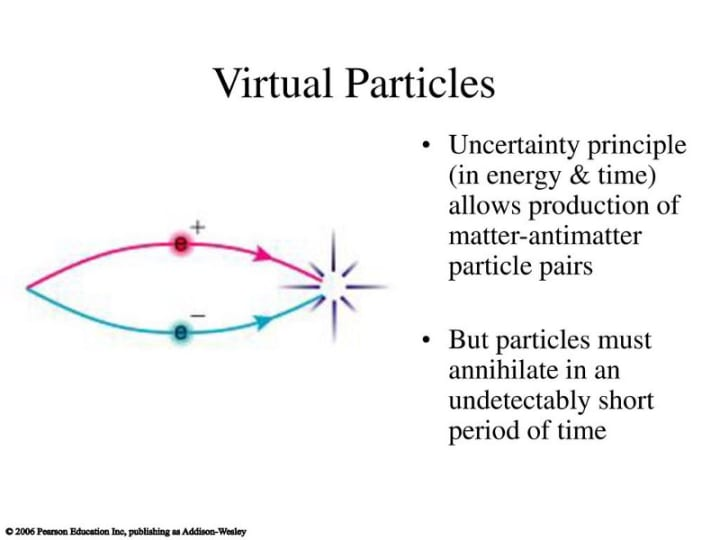It is almost as though nature has an overdraft facility. You can have something for nothing, but you have to give it back at some point. The debt, in this case, is energy, the overall energy of the system must return to zero. This occurs through particle/particle annihilation. It’s easy to see that the Heisenberg uncertainty principle underpins some of the most remarkable aspects of nature, warranting its status as perhaps the second most important result of 20th-century physics.

A version of this article was originally published by the author at Medium

scienceRobert Lea
Read next: 10 Things You Do Not Know About Kathleen Kennedy
Robert Lea

Freelance science writer/journalist. Space. Physics. Astronomy. Quantum physics. Member of the ABSW. Follow me at https://twitter.com/sciencef1rst

See all posts by Robert Lea